Slope Notes

Slope Notes

*Watch these two videos first!

https://www.youtube.com/watch?v=zihsQC0IUd8

https://www.youtube.com/watch?v=6kBN9Swe-FI

Lesson 4.1

• In the coordinate plane, the graph of x = a is a vertical line

o   What does it mean? If you are given the equation x = 4, all the points on the graph that you would draw would have a x-coordinate of 4.  (4, 0); (4, 1); (4, 2); (4, 3), etc.

o   If you plotted those points on the graph and drew a line through them, it would be vertical.

·          In the coordinate plane, the graph of y = b is a horizontal line

o   What does it mean? If you are given the equation y = 5, all the points on the graph that you would draw would have a y-coordinate of 5.  (-1, 5); (0, 5); (1, 5); (2, 5), etc.

o   If you plotted those points on the graph and drew a line through them, it would be horizontal

·        The graph of an equation involving two variables, and y, is the collection of all points (x, y) that are solutions of the equation.

• If you are asked to plot the two solution points and draw a line exactly through them, take two different numbers for x and solve for y.

For example in the equation y = 2x + 4, plug in a zero for x and solve for y. This would give you y = 2(0) + 4. Do Order of Operations next. So your one point on the line would be (0, 4). Then plug in a 1 for x and solve for y. This would give you y = 2(1) + 4. Do Order of Operations next. So your other point would be (1, 6).

• Draw a line through the two points

• Use your y = key to check your answer if you are given a calculator

Lesson 4.2

·         Slope = rise            The numerator is called the “rise”     =  the change in y

run            The denominator is called the “run”      the change in x

·         To find the slope when given 2 points,

m = y - y

x - x

o   Step 1: Label EVERYTHING

o   Step 2: Substitute numbers for letters

o   Step 3: Solve

o   Step 4: REDUCE! REDUCE! OR SIMPLIFY!

·         What type of slope do I have? Positive, Negative, Zero, or Undefined

o   If it is Positive, my line will rise from left to right

o   If it is Negative, my line will fall from left to right

o   If it is Zero, I will have a horizontal line (ex: y = 3)

o   If it is Undefined, I will have a vertical line (ex: x = 4)

***Remember this rhyme:  Undefined mean vertical line

***Another way to memorize this is UV. These letters are right next to each other in the alphabet.

Lesson 4.2 Extension

• Lines that have the same slope are parallel; that is, they do not intersect

o   Here are examples:    y= 4x + 7 and y = 4x -10

• Both of these have a slope of 4, so they would be parallel.

• Lines that intersect and form right angles are perpendicular lines. Two nonvertical lines are perpendicular when their product of their slopes are -1.

• For example in the equations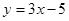and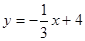, the slopes of the equations are 3 and -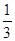. When you multiply them together, they equal -1.

Lesson 4.3

• When two quantities x and y are proportional, the relationship can be represented by the direct variation equation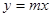, where m is the constant of proportionality. The graph ofis a line with a slope of m that passes through the origin

• If you are given a table and asked whether it is proportional, look to see if you a constant rate of change (slope) or you can work backwards in the table towards 0.

• For example in the table below, this would be proportional:

 x 4 8 12 16 y 1 2 3 4

Lesson 4.4

• Slope-intercept form :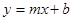y = 2nd number of the coordinate that satisfies the equation

m = the slope

x = the 1st number of the coordinate that satisfies the equation

b = the y-intercept

• The x-intercept is the point where the line crosses the x-axis

• To find the x-intercept of the line, plug in a 0 for y and solve for x. Here is an example:

o   2x + 3y = 6

o   2x + 3(0) = 6

o   2x = 6

o   x = 3

• Your ordered pair is (3, 0). You would then plot this point on the graph.

• The y-intercept is the point where the line crosses the y-axis

• To find the y-intercept of the line, plug in a 0 for x and solve for y. Here is an example:

• o   2x + 3y = 6

• o   2(0) + 3y = 6

• o   3y = 6

• o   y = 2

• o   Your ordered pair (0, 2). You would then plot this point on the graph.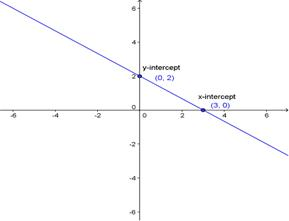• You may also use the “cover up method” where you place your hand other the x or y term and then solve for the other letter.

• Now you have the 2 points that are on the graph of the equation. After you plotted both the x-intercept and y-intercept., connect them with a straight line with arrows at the end of them. REMEMBER THAT ALL YOU NEED IS 2 POINTS TO CREATE A LINE. The easiest 2 points to use are the x-intercept and y-intercept because anything times zero equals zero!

A COUPLE OF THINGS TO REMEMBER:

• Always look to the left of the number. For example in the equation,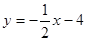, the slope is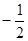and the y-intercept is -4

• DO NOT WRITE ZERO IN THE EQUATION! For example in the equation,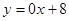, we do  not put 0x. The equation should be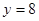. In addition, in the equation,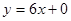, we do not put + 0. The equation should be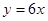• Remember the rule: What you do to one side, you must do to the other.

Lesson 4.5

·       How do I change the linear equation from standard form to slope intercept form?

o   If the equation looks like this:

Ax + By = C

o   Option #1:

o   Let’s say you had an equation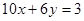o   Your goal is to make it look likeo   First, subtract 10x from both sides (inverse operation).

This would give you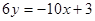o   Second, divide both sides by 6 (inverse operation).

This would give you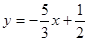This is the answer!

o   Option #2:

o   To find the slope (m) =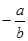o   To find the y-intercept (b) =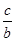o   Here’s an example: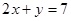m = -2

b = 7

So your equation written in slope-intercept form is: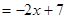• How do I graph the linear equation from standard form?

o   Use Option 1 or Option #2. Both are in slope-intercept form

o   Use Option #3:

o   Use the x-intercept and the y-intercept and draw the line

Lesson 4.6

·             Using a graph to write an equation of a line

o   Find the y-intercept from the line

o   Find another point on the line (preferably the x-intercept)

Calculate slope: m = y₂ - y₁

x₂ - x₁

o   Look at the line to check if the calculated slope is the same (positive, negative, zero, or undefined)

o   Write in slope-intercept form

·         Writing an equation of a line that passes through two points

o   Use slope-intercept form

o   Substitute for x and y with the point given

o   Substitute for m

o   Solve for b (the y-intercept)

o   Now, you know the slope (which was given to you) and the y-intercept

o   Re-write in slope-intercept form

o   Check with y=key Write an equation in slope-intercept form

o   Here is an example:

§  Write an equation of the line that passes through the point (-3, 6) with a slope of 2.

·         y = mx + b

·         Substitute 2 for m, -3 for x, and 6 for y

·         6 = 2(-3) + b

·         6 = -6 + b (Simplified)

·         Solve for b

·         12 = b

·         Re-write in slope intercept form

·         y = 2x + 12

·         If you go back and plug in (-3, 6), the left side should equal the right side!